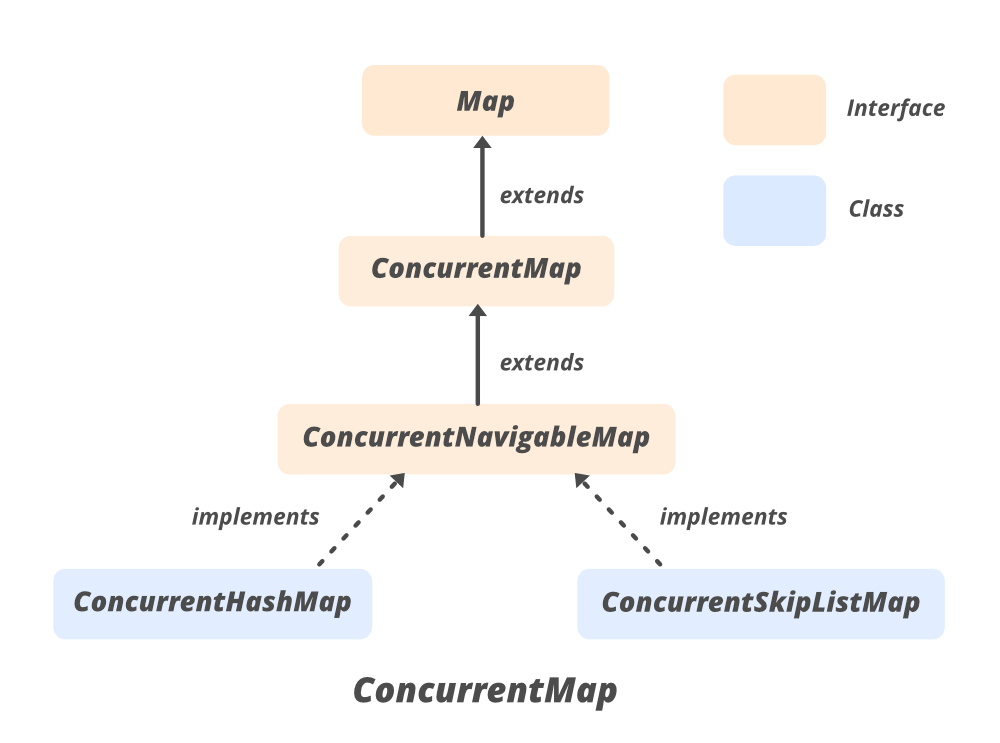Open in App
Not now

# ConcurrentMap Interface in java

• Difficulty Level : Easy
• Last Updated : 17 Sep, 2020

ConcurrentMap is an interface and it is a member of the Java Collections Framework, which is introduced in JDK 1.5 represents a Map that is capable of handling concurrent access to it without affecting the consistency of entries in a map. ConcurrentMap interface present in java.util.concurrent package. It provides some extra methods apart from what it inherits from the SuperInterface i.e. java.util.Map. It has inherited the Nested Interface Map.Entry<K, V>

HashMap operations are not synchronized, while Hashtable provides synchronization. Though Hashtable is a thread-safe, it is not very efficient. To solve this issue, the Java Collections Framework introduced ConcurrentMap in Java 1.5.

The Hierarchy of ConcurrentMapDeclaration:

```public interface ConcurrentMap<K,V> extends Map<K,V>
```

Here, K is the type of key Object and V is the type of value Object.

### Implementing Classes

Since it belongs to java.util.concurrent package, we must import is using

```import java.util.concurrent.ConcurrentMap
or
import java.util.concurrent.*
```

The ConcurrentMap has two implementing classes which are ConcurrentSkipListMap and ConcurrentHashMap. The ConcurrentSkipListMap is a scalable implementation of the ConcurrentNavigableMap interface which extends ConcurrentMap interface. The keys in ConcurrentSkipListMap are sorted by natural order or by using a Comparator at the time of construction of the object. The ConcurrentSkipListMap has the expected time cost of log(n) for insertion, deletion, and searching operations. It is a thread-safe class, therefore, all basic operations can be accomplished concurrently.

Syntax:

```// ConcurrentMap implementation by ConcurrentHashMap
CocurrentMap<K, V> numbers = new ConcurrentHashMap<K, V>();

// ConcurrentMap implementation by ConcurrentSkipListMap
ConcurrentMap< ? , ? > objectName = new ConcurrentSkipListMap< ? , ? >();
```

Example:

## Java

 `// Java Program to illustrate methods``// of ConcurrentMap interface``import` `java.util.concurrent.*;`` ` `class` `ConcurrentMapDemo {`` ` `    ``public` `static` `void` `main(String[] args)``    ``{``        ``// Since ConcurrentMap is an interface,``        ``// we create instance using ConcurrentHashMap``        ``ConcurrentMap m = ``new` `ConcurrentHashMap();``        ``m.put(``100``, ``"Geeks"``);``        ``m.put(``101``, ``"For"``);``        ``m.put(``102``, ``"Geeks"``);`` ` `        ``// Here we cant add Hello because 101 key``        ``// is already present``        ``m.putIfAbsent(``101``, ``"Hello"``);`` ` `        ``// We can remove entry because 101 key``        ``// is associated with For value``        ``m.remove(``101``, ``"For"``);`` ` `        ``// Now we can add Hello``        ``m.putIfAbsent(``101``, ``"Hello"``);`` ` `        ``// We can replace Hello with For``        ``m.replace(``101``, ``"Hello"``, ``"For"``);``        ``System.out.println(``"Map contents : "` `+ m);``    ``}``}`

Output

`Map contents : {100=Geeks, 101=For, 102=Geeks}`

### Basic Methods

The put() method of ConcurrentSkipListMap is an in-built function in Java which associates the specified value with the specified key in this map. If the map previously contained a mapping for the key, the old value is replaced.

## Java

 `// Java Program to demonstrate adding``// elements`` ` `import` `java.util.concurrent.*;`` ` `class` `AddingElementsExample {``    ``public` `static` `void` `main(String[] args)``    ``{``        ``// Instantiate an object``        ``// Since ConcurrentMap``        ``// is an interface so We use``        ``// ConcurrentSkipListMap``        ``ConcurrentMap mpp = ``new` `ConcurrentSkipListMap();`` ` `        ``// Adding elements to this map``          ``// using put() method``        ``for` `(``int` `i = ``1``; i <= ``5``; i++)``            ``mpp.put(i, i);`` ` `        ``// Print map to the console``        ``System.out.println(``"After put(): "` `+ mpp);``    ``}``}`

Output

`After put(): {1=1, 2=2, 3=3, 4=4, 5=5}`

2. Remove Elements

The remove() method of ConcurrentSkipListMap is an in-built function in Java which removes the mapping for the specified key from this map. The method returns null if there is no mapping for that particular key. After this method is performed the size of the map is reduced.

## Java

 `// Java Program to demonstrate removing``// elements`` ` `import` `java.util.concurrent.*;`` ` `class` `RemovingElementsExample {``    ``public` `static` `void` `main(String[] args)``    ``{``        ``// Instantiate an object``        ``// Since ConcurrentMap``        ``// is an interface so We use``        ``// ConcurrentSkipListMap``        ``ConcurrentMap mpp = ``new` `ConcurrentSkipListMap();`` ` `        ``// Adding elements to this map``        ``// using put method``        ``for` `(``int` `i = ``1``; i <= ``5``; i++)``            ``mpp.put(i, i);`` ` `        ``// remove() mapping associated``        ``// with key 1``        ``mpp.remove(``1``);`` ` `        ``System.out.println(``"After remove(): "` `+ mpp);``    ``}``}`

Output

`After remove(): {2=2, 3=3, 4=4, 5=5}`

3. Accessing the Elements

We can access the elements of a ConcurrentSkipListMap using the get() method, the example of this is given below.

## Java

 `// Java Program to demonstrate accessing``// elements`` ` `import` `java.util.concurrent.*;`` ` `class` `AccessingElementsExample {`` ` `    ``public` `static` `void` `main(String[] args)``    ``{`` ` `        ``// Instantiate an object``        ``// Since ConcurrentMap``        ``// is an interface so We use``        ``// ConcurrentSkipListMap``        ``ConcurrentMap chm = ``new` `ConcurrentSkipListMap();`` ` `        ``// insert mappings using put method``        ``chm.put(``100``, ``"Geeks"``);``        ``chm.put(``101``, ``"for"``);``        ``chm.put(``102``, ``"Geeks"``);``        ``chm.put(``103``, ``"Contribute"``);`` ` `        ``// Displaying the HashMap``        ``System.out.println(``"The Mappings are: "``);``        ``System.out.println(chm);`` ` `        ``// Display the value of 100``        ``System.out.println(``"The Value associated to "``                           ``+ ``"100 is : "` `+ chm.get(``100``));`` ` `        ``// Getting the value of 103``        ``System.out.println(``"The Value associated to "``                           ``+ ``"103 is : "` `+ chm.get(``103``));``    ``}``}`

Output

```The Mappings are:
{100=Geeks, 101=for, 102=Geeks, 103=Contribute}
The Value associated to 100 is : Geeks
The Value associated to 103 is : Contribute```

4. Traversing

We can use the Iterator interface to traverse over any structure of the Collection Framework. Since Iterators work with one type of data we use Entry< ? , ? > to resolve the two separate types into a compatible format. Then using the next() method we print the elements of the ConcurrentSkipListMap.

## Java

 `import` `java.util.concurrent.*;``import` `java.util.*;`` ` `public` `class` `TraversingExample {`` ` `    ``public` `static` `void` `main(String[] args)``    ``{`` ` `        ``// Instantiate an object``        ``// Since ConcurrentMap``        ``// is an interface so We use``        ``// ConcurrentSkipListMap``        ``ConcurrentMap chmap = ``new` `ConcurrentSkipListMap();`` ` `        ``// Add elements using put()``        ``chmap.put(``8``, ``"Third"``);``        ``chmap.put(``6``, ``"Second"``);``        ``chmap.put(``3``, ``"First"``);``        ``chmap.put(``11``, ``"Fourth"``);`` ` `        ``// Create an Iterator over the``        ``// ConcurrentSkipListMap``        ``Iterator > itr``            ``= chmap.entrySet().iterator();`` ` `        ``// The hasNext() method is used to check if there is``        ``// a next element The next() method is used to``        ``// retrieve the next element``        ``while` `(itr.hasNext()) {``            ``ConcurrentSkipListMap``                ``.Entry entry``                ``= itr.next();``            ``System.out.println(``"Key = "` `+ entry.getKey()``                               ``+ ``", Value = "``                               ``+ entry.getValue());``        ``}``    ``}``}`

Output

```Key = 3, Value = First
Key = 6, Value = Second
Key = 8, Value = Third
Key = 11, Value = Fourth```

### Methods of ConcurrentMap

• K – The type of the keys in the map.
• V – The type of values mapped in the map.

### Methods inherited from interface java.util.Map

My Personal Notes arrow_drop_up